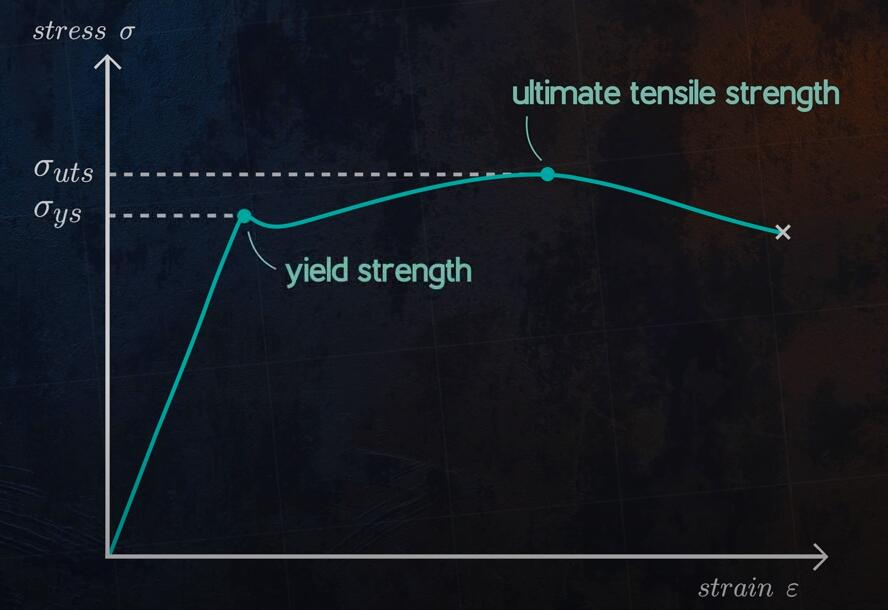> > Stiffness vs Strength, How to Differentiate Them – Difference Between Strength and Stiffness | CNCLATHING
> > Stiffness vs Strength, How to Differentiate Them – Difference Between Strength and Stiffness | CNCLATHING

# Stiffness vs Strength, How to Differentiate Them – Difference Between Strength and Stiffness | CNCLATHING

2021.5.18

Stiffness and strength are crucial properties when determining the application of materials, they would be often confused, but these two terms are not interchangeable. What’s the difference between strength and stiffness of a material? Let’s get into the distinguish between stiffness and strength.

## What is Strength?

Strength is a measure of the amount of stress that a material can take before it undergoes plastic deformation, and in that case, you are talking about the yield strength, usually, you have an upper yield and a lower yield, what is the maximum amount of stress that material can take before it breaks? That’s the ultimate tensile strength. If the applied stress is less than the yield strength, the material returns to its original shape when the stress is removed, if the applied stress exceeds the yield strength, plastic or permanent deformation occurs, and the material can no longer return to its original shape once the load is removed.

## What is Stiffness?

Stiffness is a measure of the amount of deflection that a load causes in a material. Engineers use a value called Young’s modulus, E, for stiffness. It refers to the material’s ability to return to its original form after being placed an external force, including bending, stretching, and other forms of strain. The stiffness is related to how the object bends under the load, and it can still restore its original shape after the load is removed. Stiffness is technically defined as the force per unit deformation. This deformation or displacement depends on the property of the material and the magnitude of the applied force. If one structural element deforms more than the other under the same force, its stiffness is smaller than the other.

## Stiffness vs Strength - Difference Between Strength and Stiffness

Stiffness and strength are two important physical properties of a material, but strength is not equal to stiffness. The strength of a material and its stiffness are not directly related. Strength is the ability of an object or material to resist deformation when force is applied, it measures the stress applied to a material before it permanently deforms (yield strength) or breaks (tensile strength). Stiffness is the ability of an object or material to withstand stress without breaking, it defines how the material bends to resist the external force while returning to its original form after the force is removed.

If we look at an example, a rubber surgical tube has low stiffness because it reflects a lot under load, but it is relatively strong, while a piece of glass filament deflects very little under load but might not carry a huge load before it breaks. A material can have high strength and low stiffness at the same time. If a metal cracks easily, it has a low strength, but if it has low stiffness, it can deflect a high load.

There are various types and measures of strength and stiffness.

Types of strength:

1. Yield strength: defined as the stress at which a material begins to deform plastically. Stressing a material beyond its yield strength will result in permanent deformation after the load is removed. Many structures and components are designed to ensure that they only deform elastically. This makes yield strength a commonly used criterion for defining failure in engineering design codes. It is possible for failure to occur at stress levels well below the yield strength if the applied load varies with time. This failure mode is called fatigue.

2. Ultimate strength: defined as the maximum stress that the material can withstand during a tensile test. For ductile materials, the yield and ultimate strengths are very similar in the tensile and compression tests, but for brittle materials, the material strength is much larger in compression than in tension.

The image is from YouTube.Types of stiffness:

1. Axial stiffness: the ratio of the axial load to axial deflection. An axial load happens when a force is applied parallel to the axis of another object.

2. Torsional stiffness: is the ratio of applied torsion moment to the angle of twist or the measure of the amount of torque that a radial shaft can sustain during its rotation in a mechanical system.